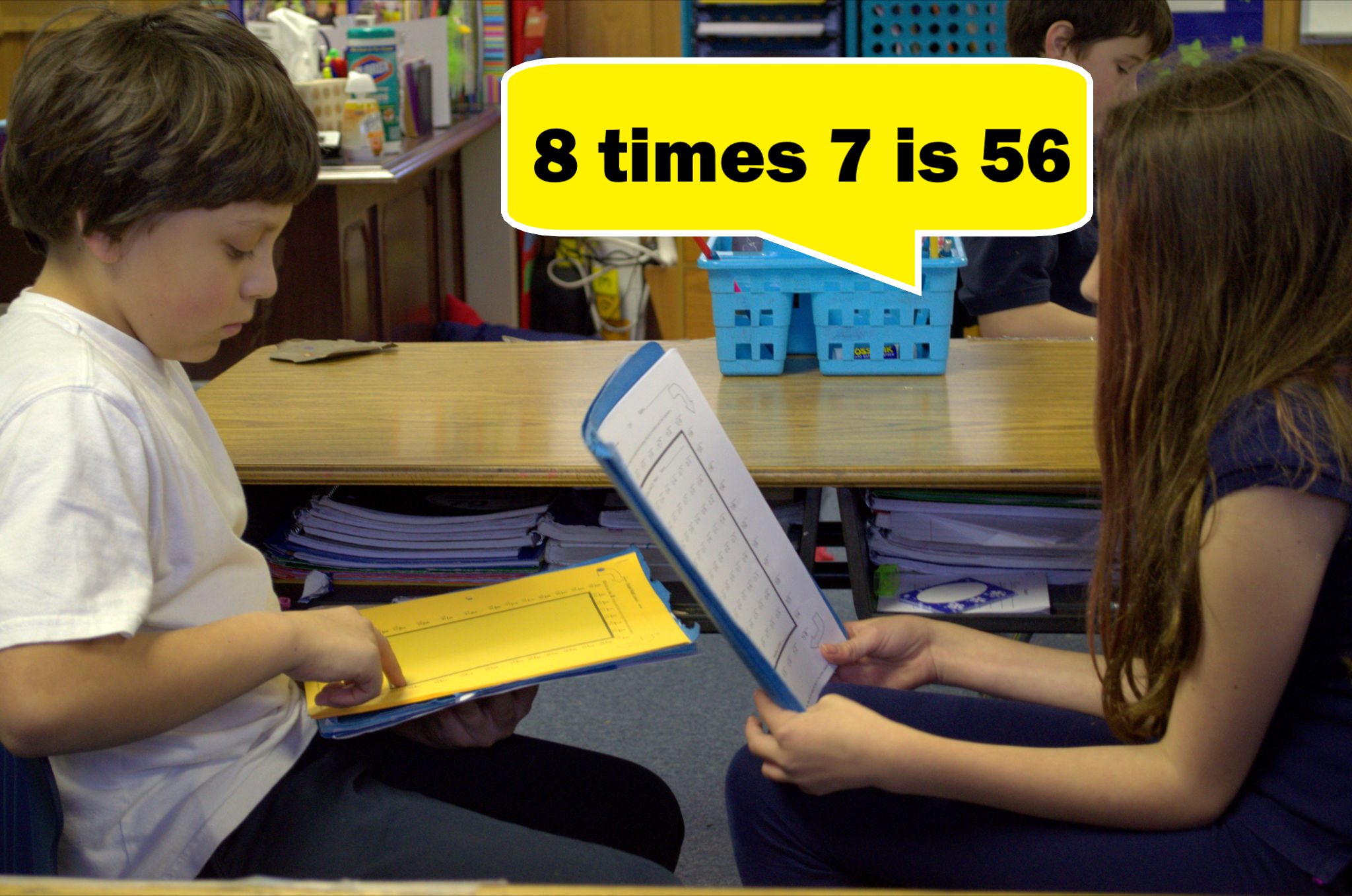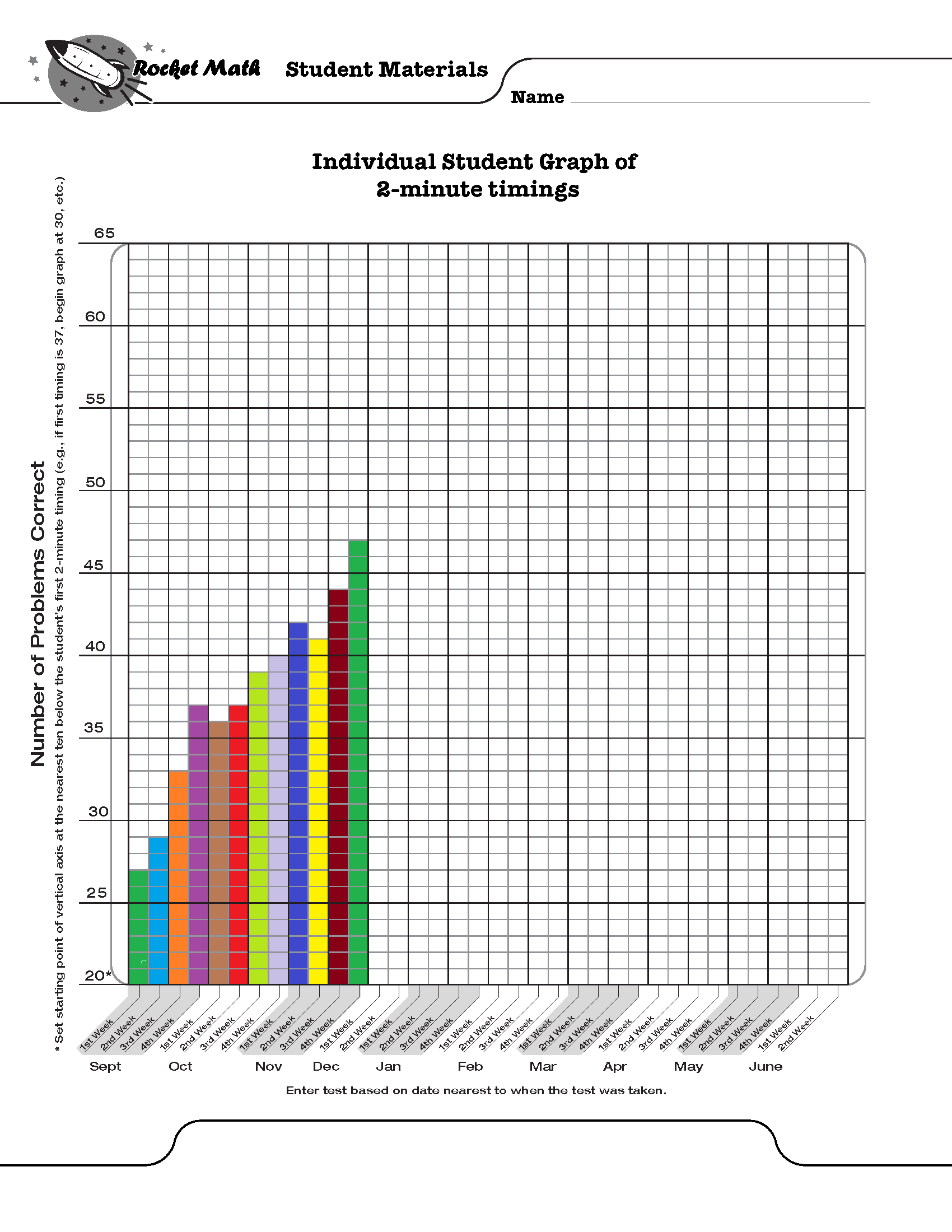## Math Fluency Programs should be part of on-going elementary school routines

Most elementary teachers do some activities to promote math fluency.  Yet many elementary children are not fluent with math facts by the time they hit upper elementary or middle school.  A hit-or-miss approach allows too many students, especially the most vulnerable, to slip through the cracks.   Math fluency programs, like Rocket Math’s Worksheet Program, need to be part of your elementary school’s routine.  Effective math fluency programs should be properly structured and every math teacher should be on board, every year.

## Math fact fluency enables students to develop number sense

Many teachers learn in their training programs about the importance of “number sense.”  Students who have “number sense” can easily and flexibly understand relations between numbers.  They can recombine numbers in various ways and see the components of numbers.  Students with number sense can intuit the fact that addition and subtraction are different ways of looking at the same relations.

What is not taught in most schools of education is that developing fluency with the basic math facts ENABLES the development of number sense much better than anything else.  Once students memorize facts, they are available for students to call upon to understand alternate configurations of numbers. Students find it much easier to see the various combinations when they when they can easily recall math facts.  Once students master the basic facts, math games that give flexibility to thinking about numbers become much easier.

It may be hard for new teachers, straight from indoctrination in the schools of education, to imagine this is true.  However, if they land in an elementary school with a strong math fact fluency program they will see the beneficial effect of memorization.

##Why is math fact fluency important

In the primary grades, students who have not developed fluency in math facts will have a harder time learning basic computation.

Students who are not fluent with math facts find the worksheets in the primary grades to be laborious work.  They finish fewer of them and may begin to dislike math for this reason.

By the time students reach upper elementary, if they have not memorized the math facts, they find it very difficult to complete math assignments at their grade level.  They find themselves unable to estimate or do mental math for problem-solving.  The need to figure out math facts will continue to distract non-fluent students while they are learning new math procedures like algorithms.

In the upper grades, their inability to figure out multiplication facts becomes a huge stumbling block.  Manipulations of fractions, decimals, and percentages will not make intuitive sense to students because they haven’t memorized those facts.  Without math fact fluency, students rarely succeed in pre-algebra and may be prevented from learning algebra and college-level math entirely.

## Math fact fluency must be assured through regular monitoringSome students will need up to ten times more practice to develop math fluency than other students.  Therefore, monitoring student success in memorizing the facts is critical. Teachers can assume that what is “enough practice” for some students is NOT going to be enough practice for all students.  Effective math fluency programs must have a progress monitoring component built in.  Progress monitoring gives comparable timed tests of all the facts at intervals during the year.  Teachers look at the results of these timed tests to check on two things:

### 1. Are students gradually improving their fluency with all the facts gradually over the year?

In other words, are students able to answer more facts in the same amount of time?  If they aren’t improving, then the instructional procedures aren’t working and need to be modified or replaced.  Math fluency programs like Rocket Math’s Worksheet Program have two minute timings of all the facts in each operation that can be given and the results graphed to see if there is steady improvement.

### 2. Are all students reaching expected levels of performance at each grade level each year?

Proper math fluency programs identify students who are not meeting expectations and give them more intensive interventions.  Ultimately, by the end of fourth grade all students should be able to fluently answer basic 1s – 9s fact problems from memory in the four operations of add, subtract, multiply and divide. Fluent performance is generally assumed to be 40 problems per minute, unless students cannot write that quickly.

Expectations vary by grade level and the sequence with which schools teach facts can vary.  While it is great to achieve all that the Common Core suggests, it is critical only to assure that students master and gain fluency in 1s through 9s facts.  Some schools in some neighborhoods may find that waiting until second grade to begin math facts may not provide enough time for all students to achieve fluency.  When to begin fact fluency and how much to expect each year should be based on experience rather than some outside dictates.

## Successful math fluency programs must have these 3 features

1. ### Sequences of small sets

No one can memorize ten similar things, like the 2s facts, all at once. Students easily master math facts when they can learn and memorize small amounts of facts at one time. Effective math fluency programs define math fact sequences, which students memorize at their pace before moving onto new math facts. Rocket Math’s fluency program uses only two facts and their reverses in each set from A through Z.

2. ### Self-paced progress

Even if you only introduce small sets of math facts, some students need more time to memorize than others.  If you introduce the facts too fast, students will begin to jumble them together and progress will be lost. The pace of introducing facts must be based on mastery—not some pre-defined pace.  This is why doing all the multiplication facts as a class in the first six weeks of third grade does not work.  It is just too fast for some students.  Once they fall behind it all becomes a blur.

3. ### Effective practice and corrections

When students are practicing facts, they will come to ones they have forgotten or can’t recall immediately.  Those are the facts on which they need more practice.  Allowing students to stop and figure out the facts they don’t know while practicing, does not help the student commit them to memory.  Instead, students need to IMMEDIATELY receive the fact and the answer, repeat it and try to remember it.  Then they need to attempt that fact again in a few seconds, after doing another couple of problems.  If they have remembered the fact and can recall it, then they are on their way to fluency.  But students must practice the next day to cement in that learning.

Math fluency programs like Rocket Math’s Worksheet Program teach students math facts in small sets, allow students to progress at their own pace, and support effective practice and error correction. Each Rocket Math Worksheet program has 26 (A to Z) worksheets specially designed to help kids gradually (and successfully) master math skills. Gain access to all of them with a Universal Subscription or just the four basics (add, subtract, multiply, divide–1s to 9s) with a Basic Subscription.